Lack-of-Fit Tests

Two goodness-of-fit tests can be requested from the PROBIT procedure: a Pearson’s chi-square test and a log-likelihood ratio chi-square test.

To compute the test statistics, you can use the AGGREGATE or AGGREGATE= option grouping the observations into subpopulations. If neither AGGREGATE nor AGGREGATE= is specified, PROC PROBIT assumes that each observation is from a separate subpopulation and computes the goodness-of-fit test statistics only for the events/trials syntax.

If the Pearson’s goodness-of-fit chi-square test is requested and the-value for the test is too small, variances and covariances are adjusted by a heterogeneity factor (the goodness-of-fit chi-square divided by its degrees of freedom) and a critical value from thedistribution is used to compute the fiducial limits. The Pearson’s chi-square test statistic is computed as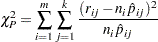where the sum onis over grouping, the sum onis over levels of response,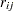is the frequency of response levelfor theth grouping,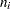is the total frequency for theth grouping, and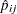is the fitted probability for theth level at theth grouping.

The likelihood ratio chi-square test statistic is computed as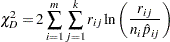This quantity is sometimes called the deviance. If the modeled probabilities fit the data, these statistics should be approximately distributed as chi-square with degrees of freedom equal to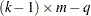, whereis the number of levels of the multinomial or binomial response,is the number of sets of independent variable values (covariate patterns), and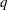is the number of parameters fit in the model.

In order for the Pearson’s statistic and the deviance to be distributed as chi-square, there must be sufficient replication within the groupings. When this is not true, the data are sparse, and the-values for these statistics are not valid and should be ignored. Similarly, these statistics, divided by their degrees of freedom, cannot serve as indicators of overdispersion. A large difference between the Pearson’s statistic and the deviance provides some evidence that the data are too sparse to use either statistic.